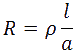# Varly loop Test For finding Location Of Faults In Underground Cables

0
878

## Varly loop Test For finding Location Of Faults In Underground Cables

Varley loop test is also for locating short-circuit and earth faults in underground cables. This test also employs the principle of the wheatstone bridge. The only difference between Murray loop test and Varley loop test is that Varley loop test provision is made for measurement of total loop resistance instead obtaining it from the relationIn this test, the ratio arms P and Q are fixed and balance position is obtained by varying the known variable resistance (Rheostat).

If the fault resistance is high, the sensitivity of Murray loop test is reduced and Varley loop test may be more suitable.

It is slightly different from Murry loop test in this the ratio arms P & Q are fixed resistance and a variable resistance (s) is connected to the test end of the faulty cable. The connection diagram for locating the earth fault is shown in fig.The key K2 is first connected to position 1, now the variable resistance S is varied till the galvanometer shows zero deflection. Let, the resistance of the variable resistance is S1 when galvanometer shows zero deflection. Then we can writeNow the switch K2 is position 2. Then the variable resistance S is varied till the galvanometer shows zero deflection. Let , the resistance of the variable resistance is S2 ,when galvanometer shows zero deflection.The value of P,Q,S1 and S2 are known , we can easily get the value of loop resistance.If the resistance of the cable per meter length is r,
Then the distance of fault form the test end isWe can easily located the short circuit fault of the under ground cable as same process as above.The circuit diagram of short circuit fault is given below:-